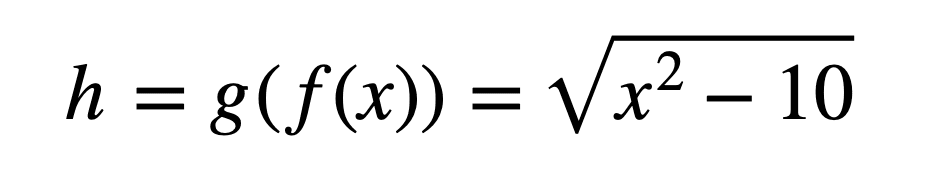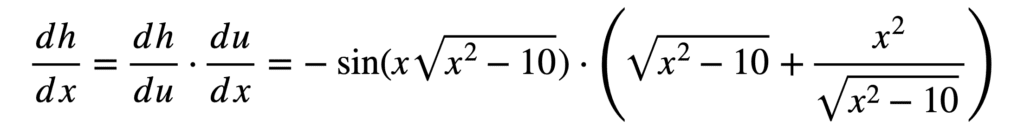# The Chain Rule of Calculus – Even More Functions

The chain rule is an important derivative rule that allows us to work with composite functions. It is essential in understanding the workings of the backpropagation algorithm, which applies the chain rule extensively in order to calculate the error gradient of the loss function with respect to each weight of a neural network. We will be building on our earlier introduction to the chain rule, by tackling more challenging functions.

In this tutorial, you will discover how to apply the chain rule of calculus to challenging functions.

After completing this tutorial, you will know:

• The process of applying the chain rule to univariate functions can be extended to multivariate ones.
• The application of the chain rule follows a similar process, no matter how complex the function is: take the derivative of the outer function first, and then move inwards. Along the way, the application of other derivative rules might be required.
• Applying the chain rule to multivariate functions requires the use of partial derivatives.

Let’s get started.

## Tutorial Overview

This tutorial is divided into two parts; they are:

• The Chain Rule on Univariate Functions
• The Chain Rule on Multivariate Functions

## Prerequisites

For this tutorial, we assume that you already know what are:

You can review these concepts by clicking on the links given above.

## The Chain Rule on Univariate Functions

We have already discovered the chain rule for univariate and multivariate functions, but we have only seen a few simple examples so far. Let’s see a few more challenging ones here. We will be starting with univariate functions first, and then apply what we learn to multivariate functions.

EXAMPLE 1: Let’s raise the bar a little by considering the following composite function:We can separate the composite function into the inner function, f(x) = x2 – 10, and the outer function, g(x) = √x = (x)1/2. The output of the inner function is denoted by the intermediate variable, u, and its value will be fed into the input of the outer function.

The first step is to find the derivative of the outer part of the composite function, while ignoring whatever is inside. For this purpose, we can apply the power rule:

dh / du = (1/2) (x2 – 10)-1/2

The next step is to find the derivative of the inner part of the composite function, this time ignoring whatever is outside. We can apply the power rule here too:

du / dx = 2x

Putting the two parts together and simplifying, we have:EXAMPLE 2: Let’s repeat the procedure, this time with a different composite function:We will again use, u, the output of the inner function, as our intermediate variable.

The outer function in this case is, cos x. Finding its derivative, again ignoring the inside, gives us:

dh / du = (cos(x3 – 1))’ = -sin(x3 – 1)

The inner function is, x3 – 1. Hence, its derivative becomes:

du / dx = (x3 – 1)’ = 3x2

Putting the two parts together, we obtain the derivative of the composite function:EXAMPLE 3: Let’s now raise the bar a little further by considering a more challenging composite function:If we observe this closely, we realize that not only do we have nested functions for which we will need to apply the chain rule multiple times, but we also have a product to which we will need to apply the product rule.

We find that the outermost function is a cosine. In finding its derivative by the chain rule, we shall be using the intermediate variable, u:

dh / du = (cos(x √(x2 – 10) ))’ = -sin(x √(x2 – 10) )

Inside the cosine, we have the product, x √(x2 – 10), to which we will be applying the product rule to find its derivative (notice that we are always moving from the outside to the inside, in order to discover the operation that needs to be tackled next):

du / dx = (x √(x2 – 10) )’ = √(x2 – 10) + x ( √(x2 – 10) )’

One of the components in the resulting term is, ( √(x2 – 10) )’, to which we shall be applying the chain rule again. Indeed, we have already done so above, and hence we can simply re-utilise the result:

( √(x2 – 10) )’ = x (x2 – 10)-1/2

Putting all the parts together, we obtain the derivative of the composite function:This can be simplified further into:

### Want to Get Started With Calculus for Machine Learning?

Take my free 7-day email crash course now (with sample code).

Click to sign-up and also get a free PDF Ebook version of the course.

## The Chain Rule on Multivariate Functions

EXAMPLE 4: Suppose that we are now presented by a multivariate function of two independent variables, s and t, with each of these variables being dependent on another two independent variables, x and y:

h = g(s, t) = s2 + t3

Where the functions, s = xy, and t = 2xy.

Implementing the chain rule here requires the computation of partial derivatives, since we are working with multiple independent variables. Furthermore, s and t will also act as our intermediate variables. The formulae that we will be working with, defined with respect to each input, are the following:From these formulae, we can see that we will need to find six different partial derivatives:We can now proceed to substitute these terms in the formulae for ∂h / ∂x and h / ∂y:And subsequently substitute for s and t to find the derivatives:EXAMPLE 5: Let’s repeat this again, this time with a multivariate function of three independent variables, $r$, $s$ and $t$, with each of these variables being dependent on another two independent variables, $x$ and $y$:
$$h=g(r,s,t)=r^2-rs+t^3$$
Where the functions, $r = x \cos y$, $s=xe^y$, and $t=x+y$.

This time round, $r$, $s$ and $t$ will act as our intermediate variables. The formulae that we will be working with, defined with respect to each input, are the following:From these formulae, we can see that we will now need to find nine different partial derivatives:Again, we proceed to substitute these terms in the formulae for ∂h / ∂x and h / ∂y:And subsequently substitute for $r$, $s$ and $t$ to find the derivatives:Which may be simplified a little further (hint: apply the trigonometric identity $2\sin y\cos y=\sin 2y$ to $\partial h/\partial y$):No matter how complex the expression is, the procedure to follow remains similar:

Your last computation tells you the first thing to do.

– Page 143, Calculus for Dummies, 2016.

Hence, start by tackling the outer function first, then move inwards to the next one. You may need to apply other rules along the way, as we have seen for Example 3. Do not forget to take the partial derivatives if you are working with multivariate functions.

This section provides more resources on the topic if you are looking to go deeper.

## Summary

In this tutorial, you discovered how to apply the chain rule of calculus to challenging functions.

Specifically, you learned:

• The process of applying the chain rule to univariate functions can be extended to multivariate ones.
• The application of the chain rule follows a similar process, no matter how complex the function is: take the derivative of the outer function first, and then move inwards. Along the way, the application of other derivative rules might be required.
• Applying the chain rule to multivariate functions requires the use of partial derivatives.

Do you have any questions?

## Get a Handle on Calculus for Machine Learning!#### Feel Smarter with Calculus Concepts

...by getting a better sense on the calculus symbols and terms

Discover how in my new Ebook:
Calculus for Machine Learning

It provides self-study tutorials with full working code on:
differntiation, gradient, Lagrangian mutiplier approach, Jacobian matrix, and much more...